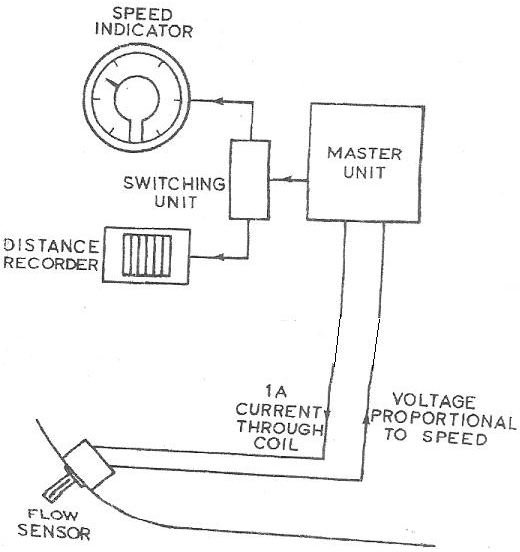# Electrical Equipments on Board Ships – The Electromagnetic Log

Page content

## Introduction

Marine engineering is not only about internal combustion or steam engines but nowadays electronics and automation play a major role in the modern vessels. In this article you will learn about a piece of equipment known as the electromagnetic log and its use on board a marine vessel.

## The EM Log

Known as EM for short, the electromagnetic log measures the speed of the ship. As you know if you want to measure the speed of a car or any other vehicle on land the simplest method is to attach a tachometer to the wheel which counts the number of revolutions and calculates the speed and distance based on that but this facility is not available on the surface of water so the EM log and the good old FM law comes to the rescue as described below.

## The Faraday Maxwell Induction Law

Basically this is not a single law but there are Maxwell’s equations and the Faraday’s law. Maxwell’s equations are actually a set of four equations which describe the behaviour of electrical and magnetic fields though I will not go into the deep analysis of these equations in this article.

Faraday’s law is derived from the Maxwell equations and speaking in the most basic terms it simply means that the change in the magnetic environment of a coil of wire will induce an emf in the wire coil. The law gives a mathematical expression of this relation.

The induced voltage in the coil is proportional to the relative speed between the magnetic field and the coil and the direction of motion.

## Application to Ship Speed Measurement

So how do we use the above formulae and principles for calculating ship speed in water? Well the answer can be seen in the diagram below which shows the EM log arrangement and as you can see the flow sensor is projected out into the sea water through the hull. The sea water acts as the conductor and generates an emf and hence a current flows in the coil which is transmitted to the bridge panel via a master unit which amplifies the signal.The signal is converted from digital to analog format for display purposes on the speed indicator unit. There is another generator which receives this signal and produces pulses whose frequency increases in proportion to the magnitude of the signal thereby giving a measurement of the distance traveled by the ship

Hence we have seen how the laws of Faraday and Maxwell help to gauge the speed and distance covered by the ship in water.

Image of EM Log System - ARSKOM Marine Company